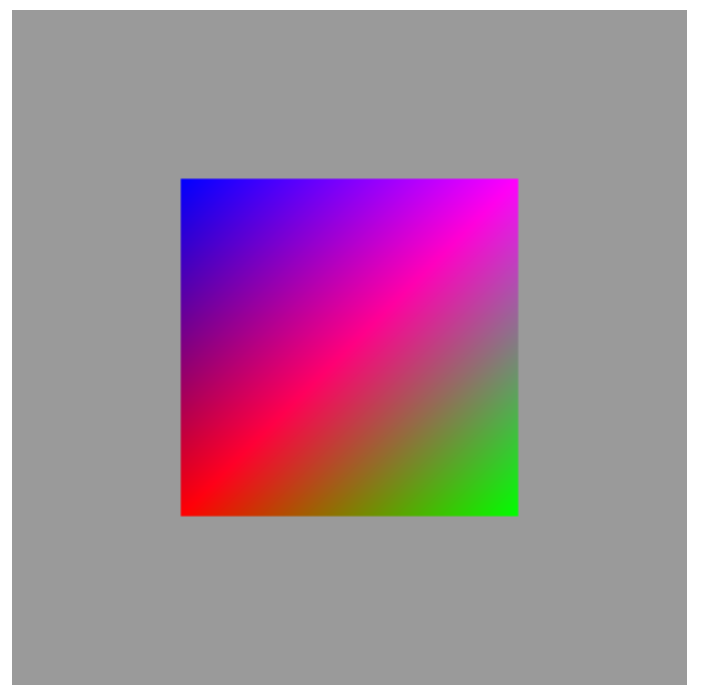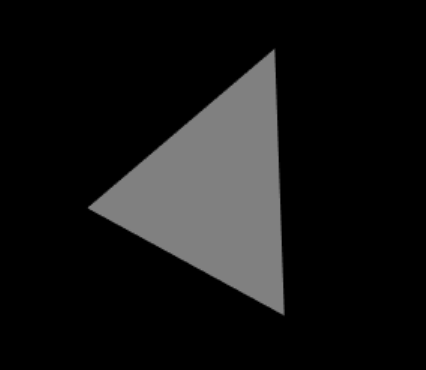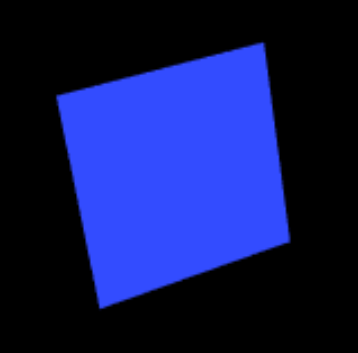# Computer Graphics

All modern displays are raster-based. A raster is a 2D matrix of pixels. In computer graphics, graphics or bitmap image is a dot matrix data structure that represents a generally rectangular grid of pixels (points of color), viewable via a monitor, paper, or other display medium. A pixel has two properties: a color and a position. Color is expressed in RGB (Red-Green-Blue) components. The position is expressed in terms of (x, y) coordinates. The origin (0, 0) is located at the top-left corner, with x-axis pointing right and y-axis pointing down.

The color values of the pixels are stored in a special part of graphics memory called frame buffer. The GPU writes the color value into the frame buffer. The display reads the color values from the frame buffer row-by-row, from left-to-right, top-to-bottom, and puts each of the values onto the screen. This is known as raster-scan. The display refreshes its screen several dozen times per second, typically 60Hz for LCD monitors and higher for CRT tubes. This is known as the refresh rate.

# Graphics Rendering Pipeline

Rendering is the process of producing image on the display from model description.The 3D Graphics Rendering Pipeline accepts data of 3D model in terms of vertices of primitives (such as point, line, triangle and quad), and produces the color-value for the pixels for the display. It consists of the following stages:

1. Vertex Processing: Process and transform individual vertices.
2. Rasterization: Convert primitives (connected vertices, e.g. line, triangle) into a set of fragments. A fragment can be treated as a pixel in 3D spaces, which is aligned with the pixel grid, with attributes such as position, color, normal and texture.
3. Fragment Processing: Process individual fragments.
4. Output Merging: Combine the fragments of all primitives (in 3D space) into 2D color-pixel for the display.

In modern GPUs, the vertex processing stage and fragment processing stage are programmable. You can write programs, known as vertex shader and fragment shader to perform your custom transform for vertices and fragments. The shader programs are written in C-like high level languages such as GLSL (OpenGL Shading Language), HLSL (High-Level Shading Language for Microsoft Direct3D), or Cg (C for Graphics by NVIDIA).

On the other hand, the rasterization and output merging stages are not programmable, but configurable - via configuration commands issued to the GPU.

# OpenGL

Open Graphics Library (OpenGL) is the premier environment for developing portable, interactive 2D and 3D graphics applications. It provides a cross-language, cross-platform application programming interface (API) for rendering 2D and 3D vector graphics. The API is typically used to interact with a graphics processing unit (GPU), to achieve hardware-accelerated rendering.

# WebGL

WebGL (Web Graphics Library) is a JavaScript API for rendering interactive 2D and 3D graphics, and it is completely controlled by the web browser. WebGL programs consist of control code written in JavaScript and shader code that is written in OpenGL Shading Language (GLSL).
The following is a demo of WebGL application:

<!doctype html>
<html>
<body>
<canvas width = "300" height = "300" id = "my_Canvas"></canvas>

<script>
/*============= Creating a canvas ==================*/
var canvas = document.getElementById('my_Canvas');
gl = canvas.getContext('experimental-webgl');

/*========== Defining and storing the geometry ==========*/

var vertices = [
-0.5,0.5,0.0,
-0.5,-0.5,0.0,
0.5,-0.5,0.0,
0.5,0.5,0.0
];

var colors = [0,0,1, 1,0,0, 0,1,0, 1,0,1,];

indices = [3,2,1,3,1,0];

// Create an empty buffer object and store vertex data
var vertex_buffer = gl.createBuffer();
gl.bindBuffer(gl.ARRAY_BUFFER, vertex_buffer);
gl.bufferData(gl.ARRAY_BUFFER, new Float32Array(vertices), gl.STATIC_DRAW);
gl.bindBuffer(gl.ARRAY_BUFFER, null);

// Create an empty buffer object and store Index data
var Index_Buffer = gl.createBuffer();
gl.bindBuffer(gl.ELEMENT_ARRAY_BUFFER, Index_Buffer);
gl.bufferData(gl.ELEMENT_ARRAY_BUFFER, new Uint16Array(indices), gl.STATIC_DRAW);
gl.bindBuffer(gl.ELEMENT_ARRAY_BUFFER, null);

// Create an empty buffer object and store color data
var color_buffer = gl.createBuffer ();
gl.bindBuffer(gl.ARRAY_BUFFER, color_buffer);
gl.bufferData(gl.ARRAY_BUFFER, new Float32Array(colors), gl.STATIC_DRAW);

/*======================= Shaders =======================*/

// vertex shader source code
var vertCode = 'attribute vec3 coordinates;'+
'attribute vec3 color;'+
'varying vec3 vColor;'+
'void main(void) {' +
' gl_Position = vec4(coordinates, 1.0);' +
'vColor = color;'+
'}';

// Create a vertex shader object
var vertShader = gl.createShader(gl.VERTEX_SHADER);

// Attach vertex shader source code
gl.shaderSource(vertShader, vertCode);

// Compile the vertex shader
gl.compileShader(vertShader);

// fragment shader source code
var fragCode = 'precision mediump float;'+
'varying vec3 vColor;'+
'void main(void) {'+
'gl_FragColor = vec4(vColor, 1.);'+
'}';

// Create fragment shader object
var fragShader = gl.createShader(gl.FRAGMENT_SHADER);

// Attach fragment shader source code
gl.shaderSource(fragShader, fragCode);

// Compile the fragmentt shader
gl.compileShader(fragShader);

// Create a shader program object to
// store the combined shader program
var shaderProgram = gl.createProgram();

// Attach a vertex shader
gl.attachShader(shaderProgram, vertShader);

// Attach a fragment shader
gl.attachShader(shaderProgram, fragShader);

// Link both the programs
gl.linkProgram(shaderProgram);

// Use the combined shader program object
gl.useProgram(shaderProgram);

/* ======== Associating shaders to buffer objects =======*/

// Bind vertex buffer object
gl.bindBuffer(gl.ARRAY_BUFFER, vertex_buffer);

// Bind index buffer object
gl.bindBuffer(gl.ELEMENT_ARRAY_BUFFER, Index_Buffer);

// Get the attribute location
var coord = gl.getAttribLocation(shaderProgram, "coordinates");

// point an attribute to the currently bound VBO
gl.vertexAttribPointer(coord, 3, gl.FLOAT, false, 0, 0);

// Enable the attribute
gl.enableVertexAttribArray(coord);

// bind the color buffer
gl.bindBuffer(gl.ARRAY_BUFFER, color_buffer);

// get the attribute location
var color = gl.getAttribLocation(shaderProgram, "color");

// point attribute to the volor buffer object
gl.vertexAttribPointer(color, 3, gl.FLOAT, false,0,0) ;

// enable the color attribute
gl.enableVertexAttribArray(color);

/*============Drawing the Quad====================*/

// Clear the canvas
gl.clearColor(0.5, 0.5, 0.5, 0.9);

// Enable the depth test
gl.enable(gl.DEPTH_TEST);

// Clear the color buffer bit
gl.clear(gl.COLOR_BUFFER_BIT);

// Set the view port
gl.viewport(0,0,canvas.width,canvas.height);

//Draw the triangle
gl.drawElements(gl.TRIANGLES, indices.length, gl.UNSIGNED_SHORT,0);
</script>
</body>
</html># LightGL

Lightgl.js provides a quickly prototype WebGL applications. Here is a simple example of lightgl.js.
.

<!DOCTYPE html>
<html><body>
<script src="./lightgl.js"></script>
<script>
var data = {
vertices: [[0, 0, 0], [1, 0, 0], [0, 1, 0]],
triangles: [[0, 1, 2]]
};

var gl = GL.create();
var angleX = 30;
var angleY = 45;
// var mesh = GL.Mesh.cube({ normals: true });
var mesh = GL.Mesh.load( data );
var offset = new GL.Vector();
var shader = new GL.Shader('\
varying vec3 normal;\
void main() {\
normal = gl_Normal;\
gl_Position = gl_ModelViewProjectionMatrix * gl_Vertex;\
}\
', '\
varying vec3 normal;\
void main() {\
gl_FragColor = vec4(normal * 0.5 + 0.5, 1.0);\
}\
');

var result;
var originalOffset;

gl.onmousedown = function(e) {
var tracer = new GL.Raytracer();
var ray = tracer.getRayForPixel(e.x, e.y);
result = GL.Raytracer.hitTestBox(tracer.eye, ray, offset.subtract(1), offset.add(1));
originalOffset = offset;
};

gl.onmousemove = function(e) {
if (e.dragging) {
if (result) {
var tracer = new GL.Raytracer();
var ray = tracer.getRayForPixel(e.x, e.y);
var t = result.hit.subtract(tracer.eye).dot(result.normal) / ray.dot(result.normal);
var hit = tracer.eye.add(ray.multiply(t));
offset = originalOffset.add(hit.subtract(result.hit));
} else {
angleY += e.deltaX;
angleX = Math.max(-90, Math.min(90, angleX + e.deltaY));
}
gl.ondraw();
}
};

gl.ondraw = function() {
gl.clear(gl.COLOR_BUFFER_BIT | gl.DEPTH_BUFFER_BIT);
gl.loadIdentity();
gl.translate(0, 0, -10);
gl.rotate(angleX, 1, 0, 0);
gl.rotate(angleY, 0, 1, 0);

// Use push and pop to guard the transform so gl is left in world space when
// gl.ondraw() ends. This way, when the GL.Raytracer reads the modelview and
// projection matrices, the rays it generates will be in world space.
gl.pushMatrix();
gl.translate(offset.x, offset.y, offset.z);
shader.draw(mesh);
gl.popMatrix();
};

gl.fullscreen();
gl.enable(gl.DEPTH_TEST);
gl.ondraw();

</script>
</body></html>

The above code looks as followingColored rectangular

<!DOCTYPE html>
<html><body>
<script src="./lightgl.js"></script>
<script>
var data = {
vertices: [[0, 0, 0], [1, 0, 0], [0, 1, 0], [1, 1, 0]],
triangles: [[0, 1, 2], [2, 1, 3]]
};

var gl = GL.create();
var angleX = 30;
var angleY = 45;
// var mesh = GL.Mesh.cube({ normals: true });
var mesh = GL.Mesh.load( data );
var offset = new GL.Vector();
var shader = new GL.Shader('\
void main() {\
gl_Position = gl_ModelViewProjectionMatrix * gl_Vertex;\
}\
', '\
uniform vec4 color;\
void main() {\
gl_FragColor = color;\
}\
');

shader.uniforms({
color: [0.2, 0.3, 1, 1]
}).draw(mesh);

var result;
var originalOffset;

gl.onmousedown = function(e) {
var tracer = new GL.Raytracer();
var ray = tracer.getRayForPixel(e.x, e.y);
result = GL.Raytracer.hitTestBox(tracer.eye, ray, offset.subtract(1), offset.add(1));
originalOffset = offset;
};

gl.onmousemove = function(e) {
if (e.dragging) {
if (result) {
var tracer = new GL.Raytracer();
var ray = tracer.getRayForPixel(e.x, e.y);
var t = result.hit.subtract(tracer.eye).dot(result.normal) / ray.dot(result.normal);
var hit = tracer.eye.add(ray.multiply(t));
offset = originalOffset.add(hit.subtract(result.hit));
} else {
angleY += e.deltaX;
angleX = Math.max(-90, Math.min(90, angleX + e.deltaY));
}
gl.ondraw();
}
};

gl.ondraw = function() {
gl.clear(gl.COLOR_BUFFER_BIT | gl.DEPTH_BUFFER_BIT);
gl.loadIdentity();
gl.translate(0, 0, -10);
gl.rotate(angleX, 1, 0, 0);
gl.rotate(angleY, 0, 1, 0);

// Use push and pop to guard the transform so gl is left in world space when
// gl.ondraw() ends. This way, when the GL.Raytracer reads the modelview and
// projection matrices, the rays it generates will be in world space.
gl.pushMatrix();
gl.translate(offset.x, offset.y, offset.z);
shader.draw(mesh);
gl.popMatrix();
};

gl.fullscreen();
gl.enable(gl.DEPTH_TEST);
gl.ondraw();

</script>
</body></html>